# Sokhotskii formulas

(diff) ← Older revision | Latest revision (diff) | Newer revision → (diff)

Formulas first discovered by Yu.V. Sokhotskii , expressing the boundary values of a Cauchy-type integral. With more complete proofs but significantly later, the formulas were obtained independently by J. Plemelj .

Let:,,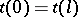, be a closed smooth Jordan curve in the complex-plane, let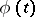be the complex density in a Cauchy-type integral along, on whichsatisfies a Hölder condition:let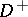be the interior of,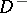its exterior, and let(1)

be a Cauchy-type integral. Then, for any pointthe limits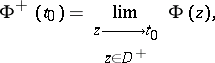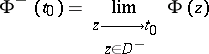exist and are given by the Sokhotskii formulas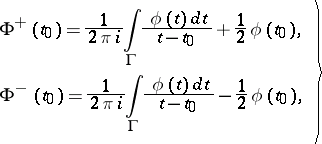(2)

or, equivalently,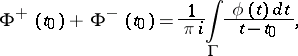The integrals alongon the right-hand sides of these formulas are understood in the sense of the Cauchy principal value and are so-called singular integrals. By taking, under these conditions,(or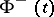) as values of the integral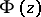on, one thus obtains a functionthat is continuous in the closed domain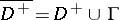(respectively,). In the large,is sometimes described as a piecewise-analytic function.

If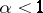, thenandare also Hölder continuous onwith the same exponent, while if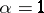, with any exponent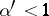. For the corner points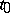of a piecewise-smooth curve(see Fig.), the Sokhotskii formulas take the form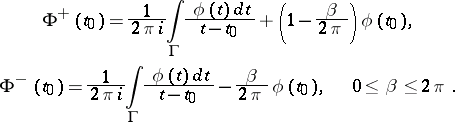(3)

In the case of a non-closed piecewise-smooth curve, the Sokhotskii formulas (2) and (3) remain valid for the interior points of the arc.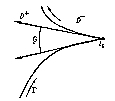Figure: s086020a

The Sokhotskii formulas play a basic role in solving boundary value problems of function theory and in the theory of singular integral equations (see , ), and also in solving various applied problems in function theory (see ).

The question naturally arises as to the possibility of extending the conditions on the contourand the density, in such a way that the Sokhotskii formulas (possibly with some restrictions) remain valid. The most significant results in this direction are due to V.V. Golubev and I.I. Privalov (see , ). For example, letbe a rectifiable Jordan curve, and let the densitybe, as before, Hölder continuous on. Then the Sokhotskii formulas (2) hold almost everywhere on, where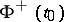andare understood as non-tangential boundary values of the Cauchy-type integral from inside and outside, respectively, but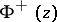and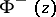are, in general, not continuous in the closed domainsand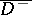.

For spatial analogues of the Sokhotskii formulas see .